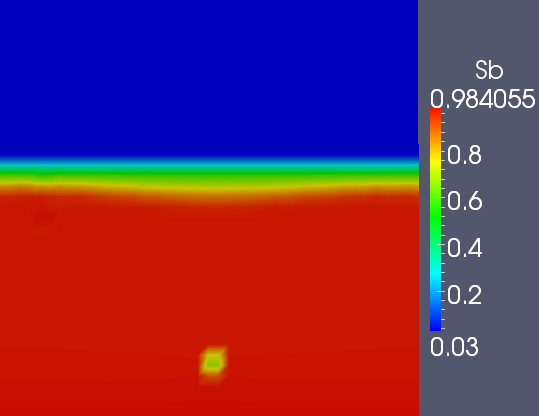# Oil production : low flow rate regime

We have a mesh 40*40 cells and the a well of 20cm*50cm.

In the following simulation when the oil saturation goes under the value 0.1 we stop the well production.

we had the following parameters :

phase a :

•  Viscosity $\mu_a=1.17\ kg/(m.s)$
• density $\rho_a=1.85 \ 10^{-5} kg/m^3$

phase b :

•  Viscosity $\mu_b=1 \ 10^{-1} kg/(m.s)$
• density $\rho_b=0.88 \ 10^3 Kg/m^3$

The initial flow rate qwell was fixed to :

•  $qwell=5 \ 10^{-5} m^3/s$

Moreover we consider that we have an isotropic domain and we fixed the permeability to :

•  $K=1 \ 10^{-9}m^2$

Here we can see the representation of the domain :

Well and domain representationThis the initial saturation that  has been taken :

Initial oil staurationSaturation in oil time=63500 sSaturation in oil time=203500 sAs the oil considered in those simulations is very viscous ( $\mu_b=1 \ 10^{-1} kg/(m.s)$ ) this decreases the mobility and it is not possible to pump with high value of the rate flow. Indeed pumping very viscous liquid with high rate flow results in a creation of a "hole in the domain" and the introduction of air in the well which disturb significantly the production. The rate flow adapted to this liquid/configuration should be around $qwell=1 \ 10^{-5} m^3/s$}.

Example of " hole" in the saturation created in the case of $qwell=5 10^{-5} \ m^3/s$ and $K=10^{-10} m^2$Indeed we can see on the previous figure that with very viscous fluid and very low permeability we cannot pump with very high flow rates.